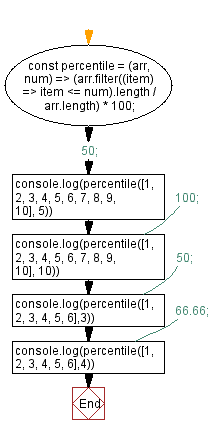# JavaScript: Calculate how many numbers in the given array are less or equal to the given value using the percentile formula

## JavaScript fundamental (ES6 Syntax): Exercise-167 with Solution

Write a JavaScript program to calculate how many numbers in the given array are less or equal to the given value using the percentile formula.

Use Array.prototype.reduce() to calculate how many numbers are below the value and how many are the same value and apply the percentile formula.

Sample Solution:

JavaScript Code:

``````const percentile = (arr, num) => (arr.filter((item) => item <= num).length / arr.length) * 100;
console.log(percentile([1, 2, 3, 4, 5, 6, 7, 8, 9, 10], 5)) // 50;
console.log(percentile([1, 2, 3, 4, 5, 6, 7, 8, 9, 10], 10)) // 100;
console.log(percentile([1, 2, 3, 4, 5, 6],3)) // 50;
console.log(percentile([1, 2, 3, 4, 5, 6],4)) // 66.66;
```
```

Sample Output:

```50
100
50
66.66666666666666
```

Flowchart:Live Demo:

See the Pen javascript-basic-exercise-167-1 by w3resource (@w3resource) on CodePen.

Improve this sample solution and post your code through Disqus

What is the difficulty level of this exercise?

Test your Programming skills with w3resource's quiz.

﻿

## JavaScript: Tips of the Day

How to check whether a string contains a substring in JavaScript?

ECMAScript 6 introduced String.prototype.includes:

```const string = "foo";
const substring = "oo";

console.log(string.includes(substring));
```

includes doesn't have Internet Explorer support, though. In ECMAScript 5 or older environments, use String.prototype.indexOf, which returns -1 when a substring cannot be found:

```var string = "foo";
var substring = "oo";

console.log(string.indexOf(substring) !== -1);
```

Ref: https://bit.ly/3fFFgZv